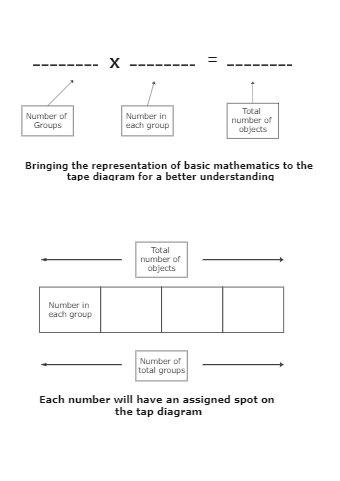Template Community / Tape diagram math problem

# Tape diagram math problemeasy diagrams
Published on 2020-09-16
Edit OnlineThe above example, ratio comparison of how much money individuals have made is represented. Tom has two boxes Jimmy has three and whereas Susan has 5 boxes. The total is \$1200. That is denoted by brackets encompassing the tape diagram. Each box/rectangle is \$120. A tape diagram is a rectangular visual model that looks like a piece of tape and is used to aid with ratio calculation. A divided bar model, fraction strip, length model, or strip diagram are other names for it. It is used to answer problems in mathematical education. A tape diagram is a rectangular visual model that looks like a piece of tape and is used to aid with ratio calculation. A divided bar model, fraction strip, length model, or strip diagram are other names for it. It is used to solve word problems in mathematics education. issues with words.
Tag
science diagram
tape diagram
math
Report
0
203PostRecommended Templates